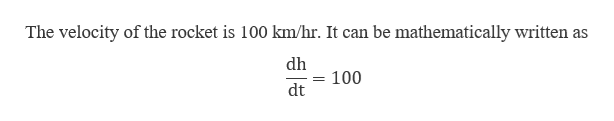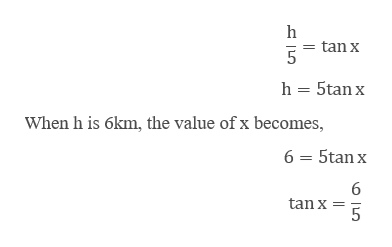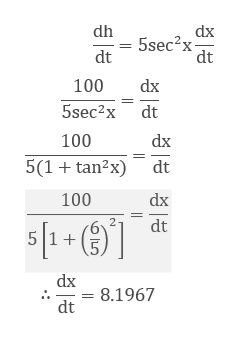# When a rocket is 6 kilometers high, it is moving vertically upward at a speed of 100 kilometers per hour. At that instant, how fast is the angle of elevation of the rocket increasing, as seen by an observer on the ground 5 kilometers from the launching pad?( Hint: The angle of elevation is the angle between the horizontal ground and the line of sight with respect to the rocket. )When the rocket is 6 kilometers high, the angle of elevation from the observer is changing at a rate of  radians per hour.

Question
34 views

When a rocket is 6 kilometers high, it is moving vertically upward at a speed of 100 kilometers per hour. At that instant, how fast is the angle of elevation of the rocket increasing, as seen by an observer on the ground 5 kilometers from the launching pad?

Hint: The angle of elevation is the angle between the horizontal ground and the line of sight with respect to the rocket. )

When the rocket is 6 kilometers high, the angle of elevation from the observer is changing at a rate of  radians per hour.

check_circle

star
star
star
star
star
1 Rating
Step 1

Let the height of the rocket be h and the angle of elevation from the observer’s eye is x.help_outlineImage TranscriptioncloseThe velocity of the rocket is 100 km/hr. It can be mathematically written as dh = 100 dt fullscreen
Step 2

As the observer is 5 km away from the launching pad, the height function in terms of x can be constructed ashelp_outlineImage Transcriptionclosetan x 5 h 5tanx When h is 6km, the value of x becomes, 6 5tan 6 tan x 5 in fullscreen
Step 3

Differentiate it with...help_outlineImage Transcriptionclosedh dx 5sec2x dt dt 100 dx 5sec2x dt 100 dx 5(1tan2x) dt 100 dx 2 dt dx 8.1967 dt - fullscreen

### Want to see the full answer?

See Solution

#### Want to see this answer and more?

Solutions are written by subject experts who are available 24/7. Questions are typically answered within 1 hour.*

See Solution
*Response times may vary by subject and question.
Tagged in
MathCalculus

### Derivative